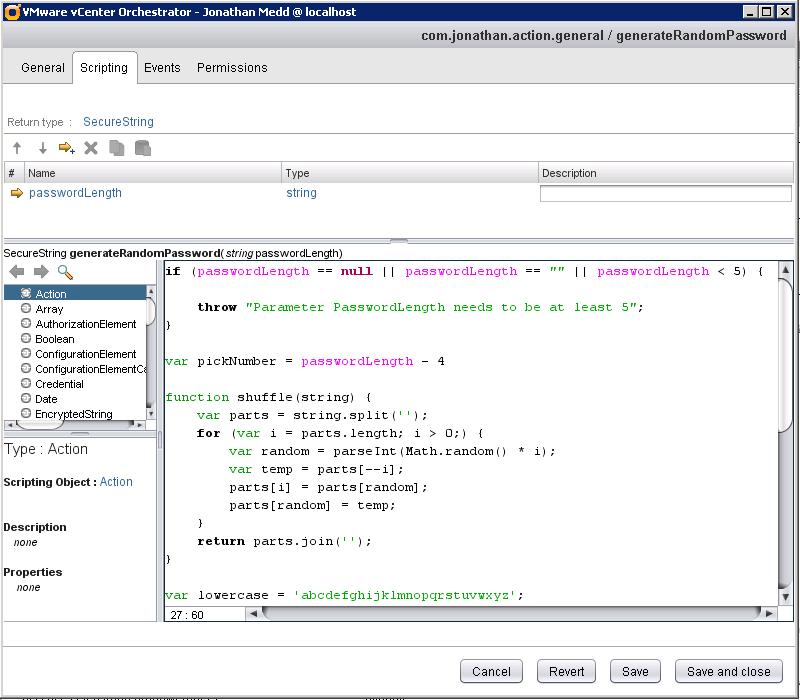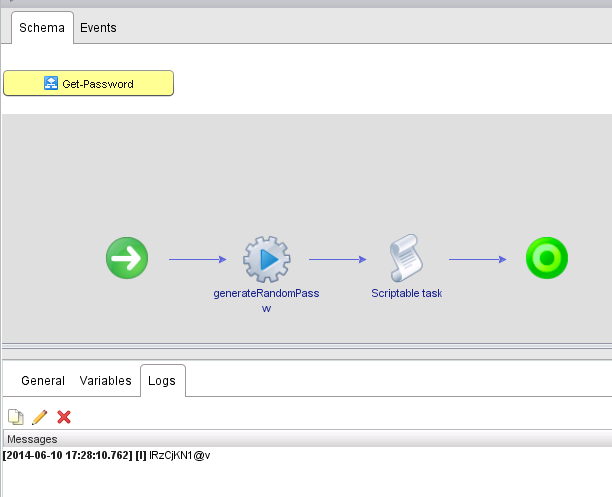# vCO Create Random Password Action

Need to create a random password in vCO, maybe to be able to create a user account in Active Directory or elsewhere? I created an action for this task which can be reused in any workflow. The code for this is below.

There’s one input passwordLength to determine how long you want the password to be.The action can be used in a workflow like so:Alternatively, you can download the action to import into your own vCO install from my vCOModules repository on GitHub, where I’m beginning to store modules of generic actions I use. Only a few items there at the minute, but plenty to follow……

[code language=“javascript”]

throw “Parameter PasswordLength needs to be at least 5”; }

var pickNumber = passwordLength - 4

function shuffle(string) { var parts = string.split(’’); for (var i = parts.length; i > 0;) { var random = parseInt(Math.random() * i); var temp = parts[–i]; parts[i] = parts[random]; parts[random] = temp; } return parts.join(’’); }

var lowercase = ‘abcdefghijklmnopqrstuvwxyz’; var uppercase = ‘ABCDEFGHIJKLMNOPQRSTUVWXYZ’; var numbers = ‘0123456789’; var special = ‘!?£\$@’; var all = lowercase + uppercase + numbers + special;

var c1 = lowercase.charAt(Math.floor(Math.random() * lowercase.length)); var c2 = uppercase.charAt(Math.floor(Math.random() * uppercase.length)); var c3 = numbers.charAt(Math.floor(Math.random() * numbers.length)); var c4 = special.charAt(Math.floor(Math.random() * special.length)); var c5 = ‘’;

for( var i=0; i < pickNumber; i++ ){ c5 += all.charAt(Math.floor(Math.random() * all.length)); }

var c6 = c1 + c2 + c3 + c4 + c5; password = shuffle(c6);